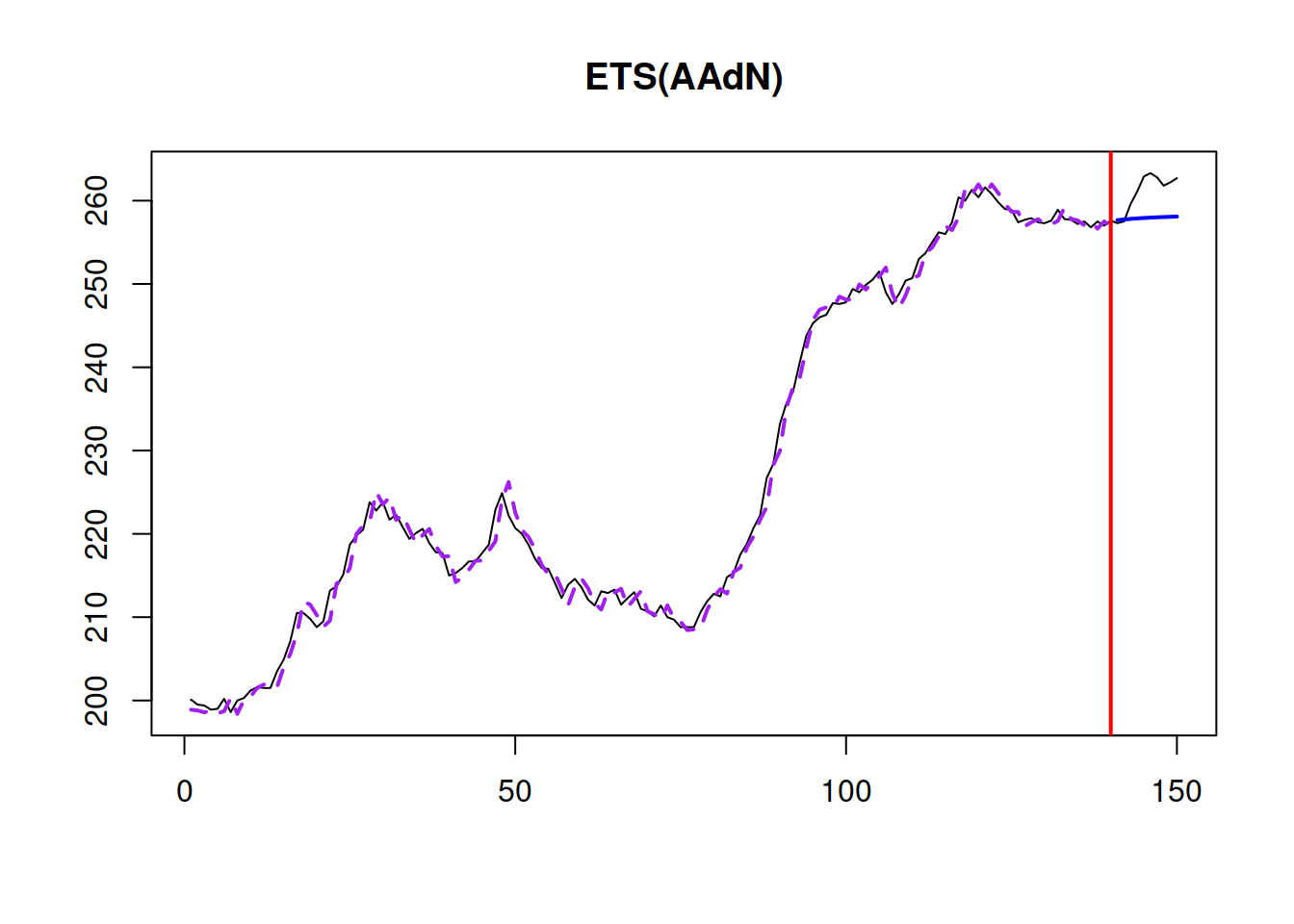This book is in Open Review. I want your feedback to make the book better for you and other readers. To add your annotation, select some text and then click the on the pop-up menu. To see the annotations of others, click the button in the upper right hand corner of the page

## 16.2 Confidence intervals for parameters

As discussed in Section 5.1 of , if several important assumptions are satisfied and CLT holds, then the distribution of estimates of parameters will follow the Normal one, which will allow us constructing confidence intervals for them. In case of ETS and ARIMA models in ADAM framework, the estimated parameters include smoothing parameters, ARMA parameters and initial values. In case of explanatory variables, the pool of parameters is increased by the coefficients for those variables. And in case of intermittent state space model, the parameters will also include the elements of the occurrence part of the model. The CLT should work if consistent estimators are used (e.g. MSE or Likelihood), if the parameters do not lie near the bounds, the model is correctly specified and moments of the distribution of error term are finite.

In case of ETS and ARIMA, the parameters are bounded and the estimates might lie near the bounds. This means that the distribution of estimates might not be normal. However, given that the bounds are typically fixed and are forced by the optimiser, the estimates of parameters will follow rectified normal distribution . This is important because knowing the distribution, we can derive the confidence intervals for the parameters. First, we would need to use t-statistics for this purposes, because we would need to estimate the standard errors of parameters. The confidence intervals will be constructed in the conventional way in this case, using the formula (see Section 5.1 of ): $\begin{equation} \mu \in (\hat{\theta_j} + t_{\alpha/2}(df) s_{\theta_j}, \hat{\theta_j} + t_{1-\alpha/2}(df) s_{\theta_j}), \tag{16.2} \end{equation}$ where $$t_{\alpha/2}(df)$$ is Student’s t-statistics for $$df=T-k$$ degrees of freedom ($$T$$ is the sample size and $$k$$ is the number of estimated parameters) and $$\alpha$$ is the significance level. Second, after constructing the intervals, we can cut their values with the bounds of parameters, thus imposing rectified distribution. An example would be the ETS(A,N,N) model, for which the smoothing parameter is typically restricted by (0, 1) region and thus the confidence interval should not go beyond these bounds as well.

In order to construct the interval, we need to know the standard errors of parameters. Luckily, they can be calculated as square roots of the diagonal of the covariance matrix of parameters (discussed in Section 16.1):

sqrt(diag(adamModelVcov))
##     alpha      beta       phi     level     trend
## 0.1144386 0.1394695 0.1256504 4.7208625 3.5642472

Based on these values and the formula (16.2) we can produce confidence intervals for parameters of any ADAM model:

confint(adamModel, level=0.99)
##            S.E.        0.5%       99.5%
## alpha 0.1144386   0.6517003   1.0000000
## beta  0.1394695   0.0000000   0.6550654
## phi   0.1256504   0.5348598   1.0000000
## level 4.7208625 190.4171720 215.0739522
## trend 3.5642472 -11.7130838   6.9027646

In order to have the bigger picture, we can produce the summary of the model, which will include the table above:

summary(adamModel, level=0.99)
##
## Response variable: BJsales
## Distribution used in the estimation: Normal
## Loss function type: likelihood; Loss function value: 241.078
## Coefficients:
##       Estimate Std. Error Lower 0.5% Upper 99.5%
## alpha   0.9507     0.1144     0.6517      1.0000 *
## beta    0.2911     0.1395     0.0000      0.6551
## phi     0.8632     0.1257     0.5349      1.0000 *
## level 202.7529     4.7209   190.4172    215.0740 *
## trend  -2.3996     3.5642   -11.7131      6.9028
##
## Sample size: 140
## Number of estimated parameters: 6
## Number of degrees of freedom: 134
## Information criteria:
##      AIC     AICc      BIC     BICc
## 494.1560 494.7876 511.8058 513.3664

The output above shows the estimates of parameters and their 99% confidence intervals. For example, based on this output we can conclude that the uncertainty about the estimate of the initial trend is very big, and in the “true model” it could be either positive or negative (or even close to zero). At the same time, the “true” parameter of the initial level will lie in 99% of the cases between 194.56 and 208.06. Just as a reminder, here how the model fit looks for ADAM on this data:

plot(adamModel,7)As another example, we can have a similar summary for ARIMA models in ADAM:

adamModelARIMA <- adam(BJsales, "NNN", h=10, holdout=TRUE,
order=list(ar=3,i=2,ma=3,select=TRUE))
summary(adamModelARIMA)
##
## Model estimated using auto.adam() function: ARIMA(0,2,2)
## Response variable: BJsales
## Distribution used in the estimation: Normal
## Loss function type: likelihood; Loss function value: 243.2819
## Coefficients:
##              Estimate Std. Error Lower 2.5% Upper 97.5%
## theta1     -0.7515     0.0830    -0.9156     -0.5875 *
## theta2     -0.0109     0.0956    -0.1998      0.1780
## ARIMAState1 -200.1902     1.9521  -204.0508   -196.3321 *
## ARIMAState2 -200.2338     2.7554  -205.6830   -194.7879 *
##
## Sample size: 140
## Number of estimated parameters: 5
## Number of degrees of freedom: 135
## Information criteria:
##      AIC     AICc      BIC     BICc
## 496.5638 497.0115 511.2720 512.3783

From the summary above, we can see that the parameter $$\theta_2$$ is close to zero, and the interval around it is wide. So, we can expect that it might change sign if the sample size increases or become even closer to zero. Given that the model above was estimated with the optimisation of initial states, we see in the summary above the values for the ARIMA states and their confidence intervals. If we used initial="backcasting", the summary would not include them.

This estimate of uncertainty via confidence intervals might also be useful to see what can happen with the estimates of parameters if the sample size increases: will they change substantially or not. If they do, then the decisions made on Monday based on the available data might differ seriously from the decisions made on Tuesday. So, in the ideal world we would want to have as lower confidence intervals as possible.

### References

• Svetunkov, I., 2021c. Statistics for business analytics. https://openforecast.org/sba/ (version: 01.10.2021)
• Wikipedia, 2021o. Rectified Gaussian distribution. https://en.wikipedia.org/wiki/Rectified_Gaussian_distribution (version: 2021-07-18)